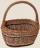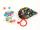Gauss

Help little C.F. Gauss sum all the integers from 1 to 400.

Result

s =  80200

Solution:Leave us a comment of example and its solution (i.e. if it is still somewhat unclear...):Be the first to comment!Next similar examples:

1. Two unknown numbersOne number is 2 less than a second number. Twice the second number is 14 less than 5 times the first. Find the two numbers.
2. SequenceWrite the first 7 members of an arithmetic sequence: a1=-3, d=6.
3. Nineteenth memberFind the nineteenth member of the arithmetic sequence: a1=33 d=5 find a19
4. Sequence 3Write the first 5 members of an arithmetic sequence: a4=-35, a11=-105.
5. 6 termsFind the first six terms of the sequence. a1 = 7, an = an-1 + 6
6. Sequence 2Write the first 5 members of an arithmetic sequence a11=-14, d=-1
7. AS sequenceIn an arithmetic sequence is given the difference d = -3 and a71 = 455. a) Determine the value of a62 b) Determine the sum of 71 members.
8. AP - simpleDetermine the first nine elements of sequence if a10 = -1 and d = 4
9. SequenceWrite the first 6 members of these sequence: a1 = 5 a2 = 7 an+2 = an+1 +2 an
10. TreesA certain species of tree grows an average of 0.5 cm per week. Write an equation for the sequence that represents the weekly height of this tree in centimeters if the measurements begin when the tree is 200 centimeters tall.
11. SeatsSeats in the sport hall are organized so that each subsequent row has five more seats. First has 10 seats. How many seats are: a) in the eighth row b) in the eighteenth row
12. SequenceBetween numbers 1 and 53 insert n members of the arithmetic sequence that its sum is 702.Determine how many apples are in baskets when in the first basket are 4 apples, and in any other is 29 apples more than the previous, and we have eight baskets.We want to prove the sentence: If the natural number n is divisible by six, then n is divisible by three. From what assumption we started?Michal said to Martin: give me one ball and I'll have twice as you. Martin said: give me 4 and we will have equally. How many balls each have?Solve a set of two equations of two unknowns: 1.5x+1.2y=0.6 0.8x-0.2y=2In six baskets, the seller has fruit. In individual baskets, there are only apples or just pears with the following number of fruits: 5,6,12,14,23 and 29. "If I sell this basket," the salesman thinks, "then I will have just as many apples as a pear." Which